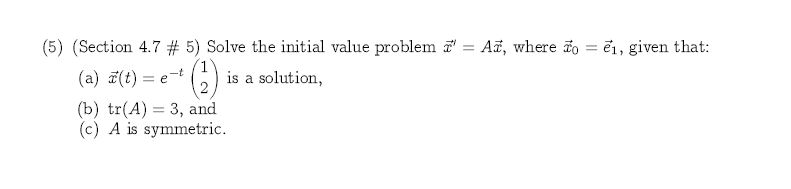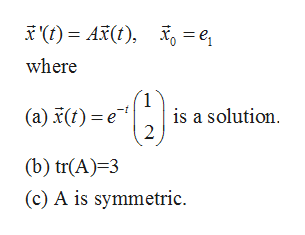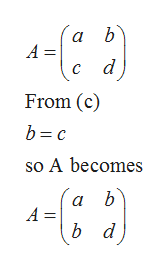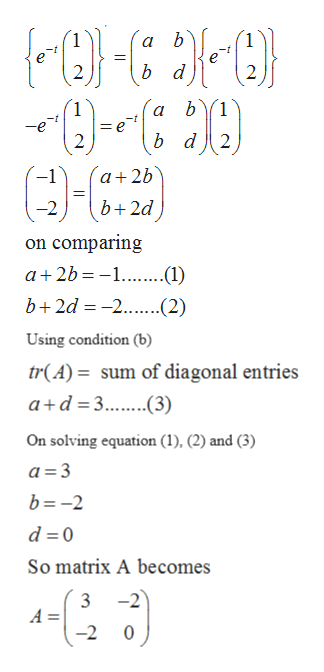# (5) (Section 4.7 #5) Solve the initial value problemAa, where o el, given that:1-t(a) (t)is a solution,= e(b) tr(A) 3, and(c) A is symmetric

Questionhelp_outlineImage Transcriptionclose(5) (Section 4.7 #5) Solve the initial value problem Aa, where o el, given that: 1 -t (a) (t) is a solution, = e (b) tr(A) 3, and (c) A is symmetric fullscreen
check_circle

Step 1

Given initial value problem ishelp_outlineImage Transcriptionclosewhere 1 (a) t) e is a solution 2 (b) tr(A)-3 (c) A is symmetric fullscreen
Step 2

Suppose A is given byhelp_outlineImage Transcriptionclosea b А — d) From (c) b c so A becomes ab A = b d fullscreen
Step 3

Now using condi...help_outlineImage Transcriptioncloseb d 2 2 1 e b d 2 2 a+2b b+2d on comparing a 2b-1...(1) b+2d -2...(2) Using condition (b) tr(A)= sum of diagonal entries a+d 3 3) On solving equation (1), (2) and (3) a 3 b=-2 d 0 So matrix A becomes 3 -2 A = -2 fullscreen

### Want to see the full answer?

See Solution

#### Want to see this answer and more?

Solutions are written by subject experts who are available 24/7. Questions are typically answered within 1 hour.*

See Solution
*Response times may vary by subject and question.
Tagged in

### Other